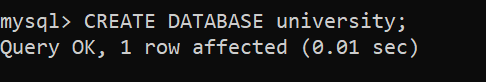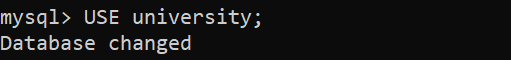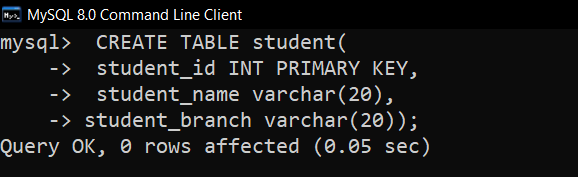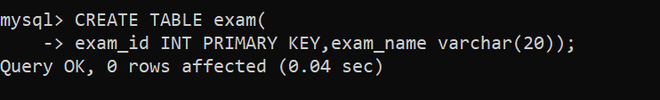GeeksforGeeks App
Open AppBrowser
Continue

# How to add a foreign key using ALTER in MySQL

In this article, we will discuss the overview of foreign keys and will discuss how to add a foreign key using ALTER in MySQL step by step. Let’s discuss it one by one.

Foreign key
If an attribute is a primary key in one table but was not used as a primary key in another table then the attribute which is not a primary key in the other table is called a foreign key. If the changes made or any data is manipulated in any of the tables the changes get reflected in both the tables with the help of foreign key constraint.

Steps to add a foreign key using ALTER in MySQL :
Here let us see how to add an attribute of student which is the primary key in the student table as a foreign key in another table exam as follows.

Step-1: Creating a database university :
Here, you will see how to create a database in MySQL as follows.

`CREATE DATABASE university;`

Output :Step-2: Using the database university :
Here, you will see how you can use the existing database which you have already created as follows.

`USE university;`

Output :Step-3: Creating a table student :
Here, you will see how to create a table in MySQL as follows.

``` CREATE TABLE student
(
student_id INT PRIMARY KEY,
student_name varchar,
student_branch varchar
);```

Output :Step-4: Viewing the description of the table :
Here, you will see how to verify the table as follows.

`DESCRIBE student;`

Output :
Here, as you can see in the description the key column of student_id is PRI which means it is the primary key in that table student.

Step-5: Creating another table exam :
In this step, you will see one more table for reference.

```CREATE TABLE exam
(
exam_id INT PRIMARY KEY,
exam_name varchar(20)
);```

Output :Step-6: Viewing the description of the table :
In this step, you can verify the table you have created.

`DESCRIBE exam;`

Output :

Step-7: Adding another column student_id into the exam table :
Here, you will see how to add another column student_id into the exam table as follows.

``` ALTER TABLE exam

Output :Step-8: Making a foreign key :
Here, you will see how to make the student_id attribute foreign key in the exam table which is the primary key in the student table as follows.

Syntax –

```ALTER TABLE table_name
REFERENCE table_name(Referencing column_name in table_name);```

Query –

```ALTER TABLE exam
REFERENCES student(student_id);```

Output :Step-9: Verifying the exam table :
Here, you will see the description of the exam table as follows.

`DESCRIBE exam;`

Output :
Now as you can see in the description of the table exam one more column student_id is added and in the Key column of description, the student_id has MUL which means it is a foreign key.

My Personal Notes arrow_drop_up